# CBSE Class 10 Science Light Reflection and Refraction Assignment Set A

Read and download free pdf of CBSE Class 10 Science Light Reflection and Refraction Assignment Set A. Get printable school Assignments for Class 10 Science. Standard 10 students should practise questions and answers given here for Chapter 10 Light Reflection And Refraction Science in Grade 10 which will help them to strengthen their understanding of all important topics. Students should also download free pdf of Printable Worksheets for Class 10 Science prepared as per the latest books and syllabus issued by NCERT, CBSE, KVS and do problems daily to score better marks in tests and examinations

## Assignment for Class 10 Science Chapter 10 Light Reflection And Refraction

Class 10 Science students should refer to the following printable assignment in Pdf for Chapter 10 Light Reflection And Refraction in standard 10. This test paper with questions and answers for Grade 10 Science will be very useful for exams and help you to score good marks

### Chapter 10 Light Reflection And Refraction Class 10 Science Assignment

Question. One light wave is incident upon a plate of refracting index m . Incident angle i , for which refractive & reflective waves are mutually perpendicular will be
(a) i = 45c
(b) i = sin-1(μ)
(c) i = cosec-1(μ)
(d) i = tan-1(μ )

Question. A perfectly reflecting mirror has an area of 1 cm2Light energy is allowed to fall on it for an hour at the rate of 10Wcm-2 . The force that acts on the mirror is
(a) 3.35 x 10-7 N
(b) 6.7 x 10-7 N
(c) 3.35 x 10-8 N
(d) 6.7 x 10-8 N

Question. An object is placed 20 cm from the concave mirror of focal length 10 cm, then image is formed at
(a) behind the mirror
(b) between the mirror and focus
(c) at focus
(d) centre of curvature of mirror

Question. A virtual image three times the size of the object is obtained with a concave mirror of radius of curvature 36 cm. The distance of the object from the mirror is
(a) 20 cm
(b) 10 cm
(c) 12 cm
(d) 5 cm

Question. An object is situated at a distance of f/2 from a convex lens of focal length f . Distance of image will be
(a) +(f/2)
(b) +(f/3)
(c) +(f/4)
(d) -f

Question. An object is placed at the centre of curvature of a concave mirror. The distance between its image and the pole is
(a) equal to f
(b) between f and 2f
(c) equal to 2f
(d) greater than 2f

Question. The refractive index of dens flint glass is 1.65 and for alcohol, it is 1.36 with respect to air, then the refractive index of the dens flint glass with respect to alcohol is
(a) 1.31
(b) 1.21
(c) 1.11
(d) 1.01

Question. Refractive index of diamond with respect to glass is 1.6. If the absolute refractive index of glass is 1.5, then the absolute refractive index of diamond is
(a) 1.4
(b) 2.4
(c) 3.4
(d) 4.4

Question. Two plane mirrors are set at right angle and a flower is placed in between the mirrors. The number of images of the flower which will be seen is
(a) One
(b) Two
(c) Three
(d) Four

Question. Velocity of light in air is 3 x 108 m/s. While its velocity in a medium is 1.5 x 108 m/s. Then, refractive index of this medium is
(a) 3
(b) 5
(c) 0.5
(d) 2

Question. A man is 6.0 ft tall. What is the smallest size plane mirror he can use to see his entire image
(a) 3.0 ft
(b) 6.0 ft
(c) 12 ft
(d) 24 ft

Question. Focal length of a plane mirror is
(a) zero
(b) infinite
(c) 25 cm
(d) -25

Question. Light waves
(a) Require air or another gas to travel through
(b) Require an electric field to travel through
(c) Require a magnetic field to travel through
(d) Can travel through perfect vacuum

Question. Which of the following lenses would you prefer to use while reading small letters found in a dictionary?
(a) A convex lens of focal length 50 cm.
(b) A concave lens of focal length 50 cm.
(c) A convex lens of focal length 5 cm.
(d) A concave lens of focal length 5 cm.

Question. Morning sun is not so hot as the mid day sun because
(a) Sun is cooler in the morning
(b) Heat rays travel slowly is the morning
(d) The sun’s rays travel a longer distance through atmosphere in the morning

Question. Where should an object be placed in front of a convex lens to get a real image of the size of the object?
(a) At the principal focus of the lens
(b) At twice the focal length
(c) At infinity
(d) Between the optical centre of the lens and its principal focus.

Question. The radius of curvature of concave mirror is 12 cm.
Then, the focal length will be
(a) 12 cm
(b) 6 cm
(c) -24 cm
(d) -6 cm

Question. An object is placed 60 cm in front of a concave mirror. The real image formed by the mirror is located 30 cm in front of the mirror. What is the object’s magnification?
(a) +2
(b) -2
(c) +0.5
(d) -0.5

Question. A spherical mirror and a thin spherical lens have each a focal length of -15 cm. The mirror and the lens are likely to be
(a) both concave
(b) both convex
(c) the mirror is concave and the lens is convex.
(d) the mirror is convex, but the lens is concave.

Question. The image of an object placed in front of a convex mirror is formed at
(a) the object itself
(b) twice the distance of the object in front of the mirror
(c) half the distance of the object in front of the mirror
(d) behind the mirror

Question. An object is placed at a distance of 10 cm in front of a plane mirror, then the distance of image from mirror will be
(a) 5 cm
(b) 10 cm
(c) 20 cm
(d) 0

Question. An object is placed 40.0 cm in front of a convex mirror. The image appears 15 cm behind the mirror. What is the focal length of the mirror?
(a) +24 cm
(b) +11 cm
(c) -11 cm
(d) -24 cm

Question. A student does the experiment on tracing the path of a ray of light passing through a rectangular glass slab for different angles of incidence. He can get a correct measure of the angle of incidence and the angle of emergence by following the labelling indicated in figure:(a) I
(b) II
(c) III
(d) IV

Question.  A student performs an experiment on finding the focal length of a convex lens by keeping a lighted candle on one end of laboratory table, a screen on its other end and the lens between them as shown in the figure. The positions of the three are adjusted to get a sharp image of the candle flame on the screen.If now the candle flame were to the replaced by a distant lamp on a far away electric pole, the student would be able to get a sharp image of this distant lamp on the screen by moving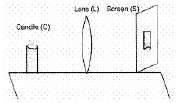(a) the screen in the direction of the lens or the lens away from the screen
(b) neither the screen nor the lens
(c) the screen in the direction of the lens or the lens in the direction of the screen
(d) the screen away fro the lens or the lens in the direction of the screen
Explanation: The candle is closer to the lens when compared to the distant lamp. Hence, the image of the candle will be farther away from the lens when compared to the image of the distant lamp. As the image will be more close to focus as the distance of the object is larger.

Question. Where should an object be placed in front of a convex lens to get a real image of the size of the object?
(a) At the principal focus of the lens
(b) At twice the focal length
(c) At infinity
(d) Between the optical centre of the lens and its principal focus.

Question. When a lemon kept in water in a bowl is viewed from outside, it appears ___________ than its actual size.
(a) None of these
(b) Smaller
(c) Larger
(d) Same
Explanation: When a lemon is kept in water in a bowl, and is viewed from the outside, it appears larger than its actual size due to refraction. When reflected rays of light travel from the lemon (which is in water) towards the eye (which is in air), the rays travel from a denser medium to a rarer medium and they bend away from the normal to the water surface.

Question. An object move a distance 'f' between 2f and f of a concave mirror. The image would have travelled a distance ofExplanation: Image of an object at 2f will be at 2f, while it will be at ∞, if object is at f.

Fill in The Blank

Question. The surface of the spoon can be approximated to a ......... mirror.

Question. In case of a rectangular glass slab, the refraction takes place at both ......... interface and .......... interface.
The emergent ray is .......... to the direction of incident ray.

Question. The degree of ......... of light rays achieved by a lens is expressed in terms of its power.

Question. The mirror used in the construction of shaving glass is .......... mirror.

Question. Light seems to travel in .........

True/False

Question. Light travels faster in glass than in air.

Question. The speed of light is different in different media.

Question. The mirror formula is valid only if the aperture of the mirror is small.

Question. Image formed by a plane mirror is always virtual and erect.

Question. A lens that is thicker at the middle than at the edge is a diverging lens.

Assertion and Reason

DIRECTION : In the following questions, a statement of assertion (A) is followed by a statement of reason (R). Mark the correct choice as:

(a) Both assertion (A) and reason (R) are true and reason (R) is the correct explanation of assertion (A).
(b) Both assertion (A) and reason (R) are true but reason (R) is not the correct explanation of assertion (A).
(c) Assertion (A) is true but reason (R) is false.
(d) Assertion (A) is false but reason (R) is true.
(e) Both Assertion and Reason are false.

Question. Assertion : For observing traffic at back, the driver mirror is convex mirror.
Reason : A convex mirror has much larger field of view than a plane mirror.

Question. Assertion : When the object moves with a velocity 2 m/s, its image in the plane mirror moves with a velocity of 4 m/s.
Reason : The image formed by a plane mirror is as far behind the mirror as the object is in front of it.

Question. Assertion : The image formed by a concave mirror is certainly real if the object is virtual.
Reason : The image formed by a concave mirror is certainly virtual if the object is real.

Question. Assertion : As light travels from one medium to another, the frequency of light does not change.
Reason : Because frequency is the characteristic of source.

Question. Assertion : An object is placed at a distance of f from a convex mirror of focal length f, its image will form at infinity.
Reason : The distance of image in convex mirror can never be infinity.

Question. Assertion : Light rays retrace their path when their direction is reversed (Law of reversibility of light rays)
Reason : For the refraction light, water is denser than air, but for the refraction of sound, water is rarer than air.

Question. Assertion : The mirror used in search lights are concave spherical.
Reason : In concave spherical mirror the image formed is always virtual.

Question. Assertion : The focal length of the convex mirror will increase, if the mirror is placed in water.
Reason : The focal length of a convex mirror of radius R is equal to, f = R/2

Question. Assertion : The refractive index of diamond is √6 and refractive index of liquid is √3 . If the light travels from diamond to the liquid, it will initially reflected when the angle of incidence is 30c.
Reason : μ = 1/sinC, where m is the refractive index of diamond with respect to liquid.

Question. Assertion : Speed of light in glass of m = 1.5 is2#108 m/ sec
Reason : According to dual theory, light has particle nature and wave nature simultaneously.

Question. Assertion : Refractive index of glass with respect to air is different for red light and violet light.
Reason : Refractive index of a pair of media depends on the wavelength of light used.

Question. Assertion : It is not possible to see a virtual image by eye.
Reason : The rays that seem to emanate from a virtual image do not in fact emanates from the image.

Question. Assertion : Plane mirror may form real image.
Reason : Plane mirror forms virtual image, if objects is real.

Question. Assertion : Concave mirrors are used as make-up mirrors.
Reason : When the face is held within the focus of a concave mirror, then a diminished image of the face is seen in the concave mirror.

Question. Assertion : As the temperature of a medium increases the refractive index decreases.
Reason : When a ray travels from vacuum to a medium, then m is known as absolute refractive index of the medium. (μvacuum = 1).

Question. Assertion : Propagation of light through an optical fibre is due to total internal reflection taking palce at the core-clade interface.
Reason : Refractive index of the material of the core of the optical fibre is greater than of air.

Question. A child is standing in front of a magic mirror. She finds the image of her head bigger, the middle portion of her body of the same size and that of the legs smaller. What will be the order of combinations for the magic mirror from the top?
Answer :  A child is standing in front of a magic mirror. Her head appears large. This means concave mirror of large focal length is used at the top. Her middle body appears of the same size in the mirror. It means plane mirror is used in the middle. Her legs appear smaller. It means convex mirror is used below plane mirror.

Question. Which type of mirror should a student use if he wants to project the image of a candle flame on the walls of the school laboratory?
Answer :  To project the image of a candle flame on the walls of the school laboratory, he should use a concave mirror as only a concave mirror forms a real image (which can be obtained on a screen) for all positions of object except when the object is placed between the pole and focus of the mirror. A convex mirror and plane mirror, on the other hand, always form virtual images.

Question. Why the glass slab must not be displaced from its boundary during the experiment to trace the path of a ray of light through a glass slab?
Answer: If the glass slab gets displaced from its original position/boundary, the angle of incidence will change accordingly and experiment will not be performed correctly. Boundary helps us to keep the glass slab in a proper position.

Question. What would be the observations of a student who performed the experiment to trace the path of a ray of light through a glass slab, whose two opposite faces are not parallel to each other?
Answer: (i) The emergent ray is not parallel to the incident ray. The direction of emergent ray depends upon the inclination of non-parallel faces.
(ii) Angles of refraction at both the faces, i.e., ∠r1 and ∠r2 are not equal.

Question. It is desired to obtain the erect image of an object, using a convex lens of focal length 20 cm.
(i) What should be range of object distance from the lens?
(ii) Will the image formed be bigger or smaller?
Answer: (i) To get the virtual and erect image formed by a convex lens, object should be kept between optical centre and focus. Therefore, range of the object distance is from 0 to 20 cm.
(ii) Enlarged and erect image is formed.

Question. What is the name given to the centre of the mirror ?
Answer :  The centre of reflecting surface of a spherical mirror is known as Pole.

Question. Define the term angle of incidence.
Answer :  The angle between an incident ray and the normal at the point of incidence is called angle of incidence.

Question. Draw ray diagram showing the image formation by a convex lens when an object is placed at twice the focal length of the lens.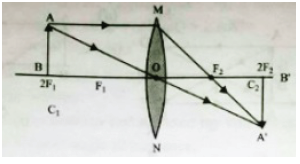Question. “A convex lens of focal length ‘F’ can form a magnified erect as well as inverted image.“ Justify this statement stating the position of the object with respect to the lens in each case for obtaining these images.
Answer: The type of image formed by a convex lens depends on the position of the object in front of the lens.
• When the object is placed between the optical centre and the focus (i.e., between O and F¢), the image formed is behind the object (on the same side). It is virtual, magnified and erect.
• When the object is placed at the focus of a convex lens, the image formed is at infinity. It is real, inverted and highly magnified.
• When the object is placed between focus and the centre of curvature (i.e., between F¢ and 2F¢), the image formed is beyond 2F. It is real, inverted and magnified.
• When the object is placed at the centre of curvature (i.e., at 2F'), the image formed is at 2F. It is real, inverted and of same size of the object.
• When the object is beyond 2F¢ (or beyond centre of curvature), the image formed is between F and 2F.
It is real, inverted and diminished.
• When the object is at infinity, the image formed is at focus. It is real, inverted and much smaller than the object (highly diminished).

Question. List two possible ways in which a concave mirror can produce a magnified image of an object placed in front of it. State the difference, if any, between these two images.
Answer: Case 1. Concave mirror can produce an erect, magnified, virtual image when the object is placed between pole and focus of the mirror
Case 2. Concave mirror also produces an inverted, magnified, real image when the object is placed between the focus and the centre of curvature.

Question. Name the type of mirror used in the following situations:
(b) Side/rear-view mirror of a vehicle
(c) Solar furnace.
Answer: (a) Concave mirror is used in the headlights of a car.
Reason. When a bulb is located at the focus of a concave mirror, the light rays get reflected from the mirror in the form of strong parallel beams. These beams travel straight over a large distance increasing visibility for safe driving.
(b) Convex mirror is used in rear/side view mirrors.
Reason. (i) It produces an erect image of the object irrespective of distance.
(ii) Image formed is diminished and gives a wide field of view of the traffic behind.
(c) Large concave mirrors are used in solar furnaces to converge the sun-rays at one point (focus) to produce heat.

Question. An object 5 cm in length is held 25 cm away from a converging lens of focal length 10 cm. Draw the ray diagram and find the position, size and the nature of the image formed.
Answer: Here h1 = 5 cm, u = –25 cm, f = +10 cm.
Using Lens formula,
1/f = 1/v - 1/u
⇒ 1/10 = 1/v - 1/-25
⇒ 1/10 - 1/25 = 1/b ⇒ 5 - 2/50 = 1/v
∴ 3/50 = 1/v ⇒ v = 50 /3 = 16.6 cm
Now v/u = h1/h2 ⇒ 50/3 / -25 = ⇒ 50/-75 = h2/5
∴ h2 = -50 x 5/75 = -10/3 = –3.3 cm

Question. A concave lens of focal length 15 cm forms an image 10 cm from the lens. How far is the object placed from the lens? Draw the ray diagram.
Answer: Here f = –15 cm, v = –10 cm, u = ?
Using Lens formula,
1/f = 1/v - 1/u ⇒ 1/-15 = 1/-10 - 1/u
1/u = -1/10 + 1/15 ⇒ 1/u = -3+ 2 /30 = -1/30
∴ u = –30 cm
∴ Object is placed at a distance of 30 cm from concave lens.

Question. “A concave mirror of focaal length ‘f ’ can form a magnified erect as well as an inverted image of an object placed in front of it.” Justify this statement stating the position of the object with respect to the mirror in each case for obtaining these images.
Answer: When the object is placed at a distance less than ‘f ’ (focal length) from the mirror, the image formed is virtual, magnified and erect.
When the object is placed at a distance of ‘f ’ (focal length) from the mirror, the image is real, inverted and highly magnified.
When the object is placed at a distance more than ‘f ’ (focal length) but less than ‘2f ’, the image formed is real, inverted and magnified.
When the object is placed at the distance of ‘2f ’ from the mirror, the image is real, inverted and same size of the object.
When the object is placed at a distance more than ‘2f ’ from the mirror, the image formed is real, inverted and diminished.

Question. An object of size 7.0 cm is placed at 27 cm in front of a concave mirror of focal length 18 cm. At what distance from the mirror should a screen be placed, so that a sharp focussed image can be obtained?
Find the size and the nature of the image.
Answer: Size of object, h = +7.0 cm u = –27 cm f = –18 cm v = ? h' = ? nature = ?
Using mirror formula
1/f = 1/v + 1/u ⇒ 1/v = 1/f - 1/u
1/v = 1/-18 - 1/-27 = -3+2/54 = -1/54 ∴ v = –54
∴ The screen should be placed at a distance of 54 cm on the object side of the mirror to obtain a sharp image.
Magnification, m = h'/h = -v/u
h'/7 = (-54)/(-27) ⇒ h' = = –2 × 7 = –14 ∴ m = h'/h = -14/7 = –2
Image size = –14 cm (–ve sign shows that image is inverted)
The image is real, inverted and enlarged.

Question. A student places a candle flame at a distance of about 60 cm from a convex lens of focal length 10 cm and focuses the image of the flame on a screen. After that he gradually moves the flame towards the lens and each time focuses the image on the screen.
(A) In which direction-toward or away from the lens, does he move the screen to focus the image?
(B) How does the size of the image change?
(C) How does the intensity of the image change as the flame moves towards the lens?
(D) Approximately for what distance between the flame and the lens, the image formed on the screen is inverted and of the same size?
Answer :  (A) As the student moves the flame towards the lens, the image is formed farther away from the focus. So, he has to move the screen away from the lens from Focus to 2F
and beyond, to focus the image. However, when object distance is less than 10 cm, no real image is formed.
(B) The size of the image gradually increases from point size to very large size.
(C) The intensity of image formed gradually decreases.
(D) Image formed on the screen will be inverted and of the same size as object when object is placed at 2F, i.e. at 20 cm (since f = 10 cm).

Question.  Two friends Kapil and Rohit were studying in the same class. One day Rohit observed that Kapil was having pain in gums during lunch time. Rohit told Kapil that his father was dentist and asked him to visit his father's clinic. Rohit's father examined Kapil with the help of a mirror and advised him not to eat too many chocolates and soft drinks. Kapil follow ed the advise of the doctor and soon he got recovered. After that he starts taking care of his mouth, as he washes his mouth properly after every meal and also starts taking a calcium rich diet. Read the given passage and answer the following questions:
i. Identify the mirror used by the dentist.
ii. Name the phenomenon of light by which doctor is able to exam ine Kapil.
iii. What values are shown by doctor, his son and Kapil?
i. The mirror used by the dentist is concave mirror.
ii. The phenomenon of light by which doctor is able to examine Kapil is reflection of light.
iii. The doctor gave the correct advise to Kapil on how to keep his mouth clean and gums healthy. The doctor’s son and doctor was helping in nature. Kapil followed the advise given by the doctor. So, he is an obedient boy.

Question. How are the images formed when an object is moved from infinity to the convex lens?
Answer : Object at Infinity. When object is at infinity, a real image is formed at F on the other side of the lens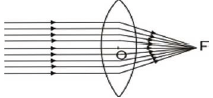Object at infinity. Image at F on the other side of lens.
However if the rays are parallel to themselves but not parallel to principal axis, then these rays after refraction will form image at focus F' and not at principal focus F.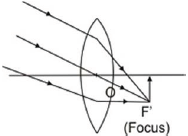Object at infinity, rays parallel to themselves but not parallel to principal axis. Image is formed at F', the focus on the other side of lens. Object beyond 2F. When the object is beyond 2F, a real, inverted, diminished image is formed between F and 2F.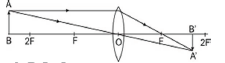Object beyond 2F, real, inverted, diminished image between F and 2F. Object at 2F.
When the object is at 2F, a real, inverted image of the same size is formed on the other side of the lens at 2F as given in Fig.Object at 2F, image at 2F on the other side of the lens. Image is of size same as that of the object. When the object is between F and 2F, its real, inverted, magnified image is formed on the other side of the convex lens as shown in fig.
Object between F and 2F real, inverted, magnified image is formed beyond 2F on the other side of lens.
Object at F. When object is placed principal focus, a real, inverted, very highly magnified image is formed at infinity.Object at F, a very highly magnified, real, inverted image is formed at infinity.
Object between F and C. When an object is placed between principal focus and optical centre of the lens, virtual, erect, magnified image is formed on the same side of the lens.Object between F and C; a virtual, erect, magnified image is formed on the same side.

Question. “A ray of light incident on a rectangular glass slab immersed in any medium emerges parallel to itself.” Draw a labelled ray diagram to justify the statement.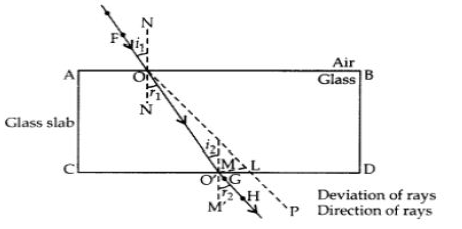Angle of incidence = 55°
Angle of refraction = 40°
Angle of emergence = 55°
FO Incident ray
GH emergent ray

Question. A concave lens of focal length 15 cm forms an image 10 cm from the lens. How far is the object placed from the lens? Draw the ray diagram.
Answer : Focal length of concave lens (OF1), f = – 15 cm
Image distance, v= – 10 cm
According to the lens formula,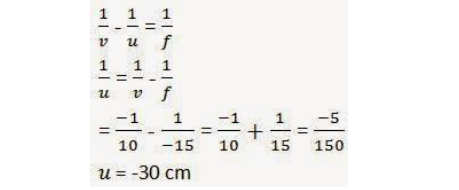The negative value of u indicates that the object is placed 30 cm in front of the lens. This is shown in the following ray diagram.Question. Draw a ray diagram to show the path of the reflected ray corresponding to an incident ray which is directed towards the principal focus of a convex mirror. Mark on it the angle of incidence and the angle of reflection.∠i = Angle of incidence
∠r = Angle of reflection

Question. Light enters from air to glass having refractive index 1.50. What is the speed of light in the glass? The speed of light in vacuum is 3 x 108 ms-1.
Answer : Refractive index of a medium, nm = Speed of light in vacuum / Speed of light in the medium
Speed of light in vacuum, c = 3 × 108 ms-1
Refractive index of glass, ng = 1.50
Speed of light in the glass, v = Speed of light in vacuum/ Refractive index of glass= c/ng=3 × 108/1.50 = 2x 108 ms-1.

Question. (a) It is desired to obtain an erect image of an object, using a concave mirror of focal length 20 cm.
(i) What should be the range of distance of the object from the mirror?
(ii) Will the image be bigger or smaller than the object?
(iii) Draw a ray diagram to show the image formation in this case.
(b) One half a convex lens of focal length 20 cm is covered with a black paper.
(i)Will the lens produce a complete image of the object?
(ii)Show the formation of image of an object placed at 2F1 of such covered lens with the help of a ray diagram.
(iii)How will the intensity of the image formed by half-covered lens compare with nn-covered lens?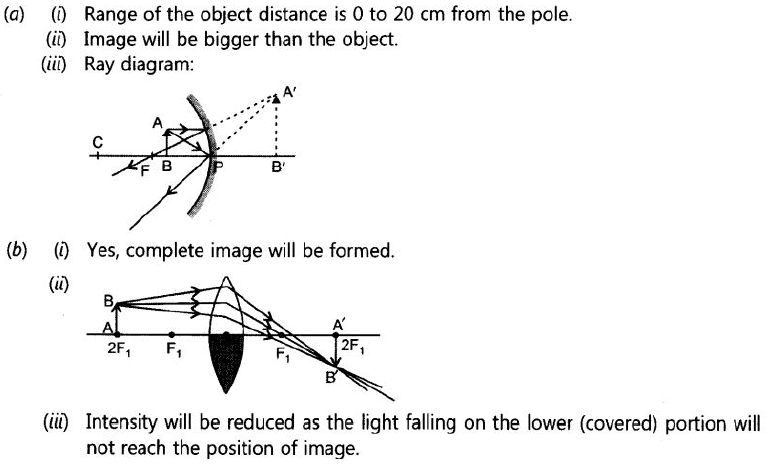CBSE Class 10 Science Chemical Reactions and Equations Assignment
 CBSE Class 10 Science Acids Bases and Salts Assignment Set A CBSE Class 10 Science Acids Bases and Salts Assignment Set B CBSE Class 10 Science Acids Bases and Salts Assignment Set C
 CBSE Class 10 Science Metals and Non Metals Assignment Set A CBSE Class 10 Science Metals and Non Metals Assignment Set B
 CBSE Class 10 Science Carbon and its compounds Assignment Set A CBSE Class 10 Science Carbon and its compounds Assignment Set B CBSE Class 10 Science Carbon and its compounds Assignment Set C CBSE Class 10 Science Carbon and its compounds Assignment Set D CBSE Class 10 Science Carbon and its compounds Assignment Set E
 CBSE Class 10 Science Periodic Classification Of Elements Assignment Set A CBSE Class 10 Science Periodic Classification Of Elements Assignment Set B CBSE Class 10 Science Periodic Classification Of Elements Assignment Set C
 CBSE Class 10 Science Biology Life Process Respiration Assignment CBSE Class 10 Science Biology Nutrition Assignment CBSE Class 10 Science Biology Respiration Assignment CBSE Class 10 Science Biology Transport Enrichment Assignment CBSE Class 10 Science Biology Transportation Assignment CBSE Class 10 Science Life Processes Assignment Set A CBSE Class 10 Science Life Processes Assignment Set B CBSE Class 10 Science Life Processes Assignment Set C
 CBSE Class 10 Science Control and Coordination Assignment Set A CBSE Class 10 Science Control and Coordination Assignment Set B CBSE Class 10 Science Control and Coordination Assignment Set C CBSE Class 10 Science Control and Coordination Assignment Set D
 CBSE Class 10 Science How do Organisms Reproduce Assignment Set A CBSE Class 10 Science How do Organisms Reproduce Assignment Set B
 CBSE Class 10 Science Heredity and Evolution Assignment Set A CBSE Class 10 Science Heredity and Evolution Assignment Set B CBSE Class 10 Science Heredity and Evolution Assignment Set C
 CBSE Class 10 Science Light Reflection and Refraction Assignment Set A CBSE Class 10 Science Light Reflection and Refraction Assignment Set B CBSE Class 10 Science Light Reflection and Refraction Assignment Set C CBSE Class 10 Science Light Reflection and Refraction Assignment Set D CBSE Class 10 Science Light Reflection and Refraction Assignment Set E CBSE Class 10 Science Light Reflection and Refraction Assignment Set F
 CBSE Class 10 Science Human Eye and Colorful World Assignment Set A CBSE Class 10 Science Human Eye and Colorful World Assignment Set B CBSE Class 10 Science Human Eye and Colorful World Assignment Set C
 CBSE Class 10 Physics Electricity Assignment Set A CBSE Class 10 Physics Electricity Assignment Set B CBSE Class 10 Physics Electricity Assignment Set C CBSE Class 10 Science Electricity Assignment Set A CBSE Class 10 Science Electricity Assignment Set B CBSE Class 10 Science Electricity Assignment Set C
 CBSE Class 10 Science Magnetic Effects of Electric Current Assignment Set A CBSE Class 10 Science Magnetic Effects of Electric Current Assignment Set B CBSE Class 10 Science Magnetic Effects of Electric Current Assignment Set C CBSE Class 10 Science Magnetic Effects of Electric Current Assignment Set D
 CBSE Class 10 Science Sources of Energy Assignment Set A CBSE Class 10 Science Sources of Energy Assignment Set B CBSE Class 10 Science Sources of Energy Assignment Set C CBSE Class 10 Science Sources of Energy Assignment Set D
 CBSE Class 10 Science Our Environment Assignment Set A CBSE Class 10 Science Our Environment Assignment Set B CBSE Class 10 Science Our Environment Assignment Set C
 CBSE Class 10 Science Sustainable Management of Natural Resources Assignment Set A CBSE Class 10 Science Sustainable Management of Natural Resources Assignment Set B CBSE Class 10 Science Sustainable Management of Natural Resources Assignment Set C
 CBSE Class 10 Physics Revision Assignment Set A CBSE Class 10 Physics Revision Assignment Set B CBSE Class 10 Physics Revision Assignment Set C CBSE Class 10 Physics Revision Assignment Set D CBSE Class 10 Physics Revision Assignment Set E CBSE Class 10 Physics Revision Assignment Set F CBSE Class 10 Physics Revision Assignment Set G CBSE Class 10 Physics Revision Assignment Set H
 CBSE Class 10 Science Assignment CBSE Class 10 Science Assignments Collection CBSE Class 10 Science Energy Crossword Puzzle Assignment
 CBSE Class 10 Physics Question Bank CBSE Class 10 Physics Tungsten Assignment
 CBSE Class 10 Science Chemistry Cleaning Capacity of Soap Assignment CBSE Class 10 Science Chemistry Determination of pH Assignment CBSE Class 10 Science Chemistry Different Types of Chemical Reactions Assignment CBSE Class 10 Science Chemistry Displacement Reaction and Reactivity Series Assignment CBSE Class 10 Science Chemistry Properties of Acetic Acid Assignment CBSE Class 10 Science Chemistry Saponification Preparation of Soap Assignment CBSE Class 10 Science Chemistry Study of properties of HCI Acid and NaOH Assignment

Tags